# A Numerical Study of Heat Transfer and Flow Characteristics of Pulsatile Blood Flow in a Tapered Artery with a Combination of Stenosis and Aneurysm

A Numerical Study of Heat Transfer and Flow Characteristics of Pulsatile Blood Flow in a Tapered Artery with a Combination of Stenosis and Aneurysm
Department of Mathematics, Technical and Vocational University (TVU), Tehran 17115-131, Iran

Department of Mathematics, Urmia University of Technology, Urmia 57155-419, Iran

Corresponding Author Email:
ah.haghighi@gmail.com
Page:
11-21
|
DOI:
https://doi.org/10.18280/ijht.370102
3 June 2018
|
Accepted:
23 December 2018
|
Published:
31 March 2019
| Citation

OPEN ACCESS

Abstract:

This research focuses on numerical patterns of temperature distribution and blood flow through tapered arteries. The blood flow is considered as an incompressible and fully developed flow with Non-Newtonian nature assumed as modified Cross fluid which is inside a body under certain acceleration. Also, the impact of heat transfer regarding a single layer model on an unstable and pulsatile blood flow through a designed tapered artery with a combination of stenotic and aneurysm is investigated. The wall of artery is designed as an elastic tube, so, its geometry changes during the numerical computations is up to time. This research uses a suitable coordinate transformation to create an exact mesh with rectangular mesh units. In the case of the thermophysical parameters of blood flow field including the velocity pattern and temperature distributions, the governing equations are managed and solved based on the finite difference method and then, the reported results are discussed as a function of velocity and temperature. The outputs of this mathematical modeling are compared with some available and proved results in previous researches to confirm the accuracy and validity of the method. The variations in dynamic features of blood flow wall shear stress, volumetric flow rate and flow resistance can be analyzed and computed on basis of the pattern of velocity profile in assumed domain. Furthermore, contour plots of temperature and velocity for specified parameters are provided.

Keywords:

body acceleration, finite difference method, heat transfer, cross fluid, stenosis and aneurysm

1. Instruction

As we know the atherosclerosis is one of the reasons for cardiovascular disease. When the arteries are narrowing because of atherosclerosis causes their capability for oxygen transmission will be decreased gradually. The high level of cholesterol in blood causes the deposition of fat on inner wall of the arteries which leads to the stenotic, narrowing and hardening problems for vessels [1-3]. Blood is a red suspension fluid composed of four main parts including the red and white blood cells, plasma and platelets [4-5]. The collaboration of scientists and engineers during the years between 1970 and 2008 showed that the atherosclerosis affects the flow characteristics inside the human body, so, the investigations were continued on the importance of dynamical factors regarding the blood flow. Most of the previous investigations assumed the blood vessel as an inelastic geometry the shape of walls are independent of time with some single or overlapping stenosis, and then the blood flow characteristics were investigated and analyzed applying the perturbation method. Also in some, the domain of blood flow is considered as a Newtonian, steady, one dimensional and axisymmetric case, consequently, these models couldn’t present the precise results because of the Non-Newtonian nature of the blood [6-10].

When the artery diameter decreases, the blood cannot flow easily at the low Newtonian shear rates, also it is pointed out in many works of literature, like , the nature of blood flow requires a non-Newtonian description to well consider the shear stress effect. For this reason, in the presented study we considered a non-Newtonian (Cross fluid) model of the blood flow. In this research we forced the body to have a certain acceleration to be closer to the real model (Travelers roller coaster, using jackhammer and etc.) compared with the previous works, in this way, a dynamical parameter is added to the simulation of blood flow [11-12]. This acceleration affects the blood flow to have some abnormal and sometimes dangerous reactions against the sudden movements which need to be investigated (loss of vision, increasing heart rate, abdominal pain, bleeding in the face) [13-15]. Zaman et al.  performed an interesting work on the unsteady blood flow inside a catheterized artery as a mild stenotic in channel using the micropolar model to clarify the rheological Factors of the blood. Considered the heat transfer effect on blood flow as another dynamical parameter. Their results are so valuable because when the heat dissipation rate is increased over than the normal situation the body cannot compensate the lost water and salt, so, the body temperature will be increased. This situation leads to some sudden problems like contraction and deterioration of the muscles [17-19]. Mekheimer et al. determined the impact of heat fluctuations on blood flow through the anisotropic ally tapered elastic artery with overlapping stenosis [20-21]. Sankar and Lee used the a sisko fluid in their work for modeling an artery with a mild stenosis, in their researches the core flow and the plasma (in peripheral layer) are adjusted as Casson and Newtonian fluids, respectively . Sarifuddin et al. introduced a numerical model for blood flow with exact and appropriate boundary conditions. It should be said that their method used a generalized Newtonian model and solved the Navier-stokes equations using Mac method . Assumed the blood can be considered as a Casson fluid. They simulated a blood vessel as a small tube and checked the heat transfer effects on the flow in the determined domain (small tube). They fund that the heat transfer rate increases on the motionless wall when the viscosity improves. Victor and Shah  conducted their numerical work on the blood flow as a two phase unsteady pulsatile flow, then they discussed the effect of acceleration on the blood flow properties. Zaman et al.  presented a numerical model on the blood flow which can handle the nonlinear continuity and momentum equations using finite difference method. Lorenzini and Casalena presented a CFD analysis for pulsatile blood flow in an atherosclerotic human artery .

The present study provides a numerical and mathematical model to evaluate the effect of heat transfer process on blood characteristics inside the artery with determined stenotic under body acceleration. The recent scientific works tried to assume some real considerations on the simulations in order to present an actual view of what happened in the blood arteries. This matter motivated us to perform an exact and comprehensive mathematical modeling on the blood arteries with actual situations (more than ever) such as heat transfer consideration, artery with elastic wall, unsteady flow, pulsatile condition and finally adjusting certain acceleration to the problem. The present study is organized in the following fashion: section2, geometry problem. Section3, describes basic equations governing the blood flow under consideration and Suitable coordinate transformation. Section4, contains the scheme for the numerical solution and investigate the stability. Section5, numerical results and discussion are presented. Section6 conclusion summary.

2. The Geomery of the Stenosis and Aneurysms

This research uses a mathematical domain the blood flow in the shape of a tapered artery with axisymmetric geometry in the presence of body acceleration. The wall of artery is modeled as an elastic cylindrical tube (circular Cross-section) which can conduct a non-Newtonian fluid as the blood. This mathematical modeling applies the cylindrical coordinate system (r,$\theta$,z) at constant temperature T. In this coordinate system r, $\theta$ and z represent the radius, angle and direction along the tube (artery), reflectively. Also, the boundaries along the tube (artery) are considered as dimensionless time-dependent geometries. The mathematical modeling of the artery is presented by figure 1 [16, 27, 28].

R(z)=\left\{ \begin{align} & (1+\xi z)(1-(\frac{\sigma }{2}(1+\cos 2\pi (z-{{\sigma }_{i}}-\frac{1}{2})))){{a}_{1}}(t), \\ & \begin{matrix} \begin{matrix} \begin{matrix} \begin{matrix} \begin{matrix} {} & {} \\ \end{matrix} & {} & {} \\ \end{matrix} & {} & {} \\ \end{matrix} & {} & {} \\ \end{matrix} & {} & {} & {} \\ \end{matrix}\sigma \le z\le \sigma +1 \\ & (1+\xi z){{a}_{1}}(t)\begin{matrix} \begin{matrix} \begin{matrix} \begin{matrix} \begin{matrix} \begin{matrix} \begin{matrix} {} & {} \\ \end{matrix} & {} \\ \end{matrix} & {} \\ \end{matrix} & {} \\ \end{matrix} & {} \\ \end{matrix} & {} \\\end{matrix} & {} \\ \end{matrix}o.w\begin{matrix} {} & {} \\ \end{matrix} \\ \end{align} \right. (1)

In this modeling $σ_i^*$  shows the critical point ${σ^*}={σ_1^*}$ is the positive critical point (regarding stenosis), ${σ_2^*}={σ_1^*}$  is the negative critical point (for aneurysms), ${{l}_{i}}$  presents the length of stenosis or aneurysms, and finally ${\xi}(=\tan{\varphi})$ introduces the tapered parameter for the area after the aneurysms.

s.t ${{l}_{0}}={{l}_{1}}={{l}_{2}} and$

${{a}_{1}}(t)=1+{{K}_{r}}\cos (\omega t-\varphi )\begin{matrix} \begin{matrix} \begin{matrix} {} & {} \\ \end{matrix} & \sigma =\frac{{{\beta }_{i}}}{{{l}_{i}}},\xi =\frac{{{\xi }^{*}}{{l}_{i}}}{a} \\ \end{matrix} & {} \\ \end{matrix}$ (2)

In this equation ω=2πfp ,   Kr and φ represent the angular frequency, oscillation parameters and phase angle, respectively.

## figure_1.pngFigure 1. Schematic model of the artery in longitudinal section

3. Formulation of the Problem

As said in the introduction, this research wants to model the blood in the vessel as an incompressible and unsteady flow. Also in the investigated model we have considered pulsatile nature of the blood flow, like as ref . The velocity components should be defined in r and z directions, so, the governing equations are as follow.

The form of dimensionless continuity equation:

$\sigma (\frac{\partial u}{\partial r}+\frac{u}{r})+\frac{\partial v}{\partial z}=0.$

The form of dimensionless Navier-Stokes equation for non-Newtonian fluid Cross [19, 20]

\begin{align} & \operatorname{Re}\sigma {{\varepsilon }^{2}}(\frac{\partial u}{\partial t}+\varepsilon (\sigma u\frac{\partial u}{\partial r}+v\frac{\partial u}{\partial z}))= \\ & \begin{matrix} {} & {} & {} \\\end{matrix}-\frac{\partial p}{\partial r}+{{\varepsilon }^{2}}(\frac{1}{r}\frac{\partial }{\partial r}(r{{S}^{rr}})+\frac{\partial }{\partial z}({{S}^{rz}})), \\\end{align} (3)

\begin{align} & \operatorname{Re}[\frac{\partial v}{\partial t}]+\operatorname{Re}(\sigma \varepsilon u\frac{\partial v}{\partial r}+\varepsilon v\frac{\partial v}{\partial z})= \\ & \begin{matrix} \begin{matrix} {} & {} \\\end{matrix} & {} \\\end{matrix}\begin{matrix} {} & {} \\\end{matrix}-\frac{\partial p}{\partial z}+G(t)+(\frac{1}{r}\frac{\partial }{\partial r}(r{{S}^{rz}})+{{\varepsilon }^{2}}\frac{\partial }{\partial z}({{S}^{zz}})) \\\end{align} (4)

\begin{align} & \operatorname{Re}{{p}_{r}}(\frac{\partial T}{\partial t}+\varepsilon (\sigma u)\frac{\partial T}{\partial r}+v\frac{\partial T}{\partial z})= \\ & \begin{matrix} {} & {{B}_{r}}(\sigma {{\varepsilon }^{2}}{{S}^{rr}}\frac{\partial u}{\partial r}+{{S}^{rz}}\frac{\partial v}{\partial r}+\sigma {{\varepsilon }^{2}}{{S}^{zr}}\frac{\partial u}{\partial z}+{{\varepsilon }^{2}}{{S}^{zz}}\frac{\partial v}{\partial z}) \\\end{matrix} \\ & \begin{matrix} {} & {} \\\end{matrix}+(\frac{{{\partial }^{2}}T}{\partial {{r}^{2}}}+\frac{1}{r}\frac{\partial T}{\partial r}+{{\varepsilon }^{2}}\frac{{{\partial }^{2}}T}{\partial {{z}^{2}}}) \\\end{align} (5)

The components of sij extra stress are provided as bellow

${{S}^{rz}}=(M+(1-M)S)(\frac{\partial v}{\partial r}+\sigma \frac{\partial u}{\partial z}),$

${{S}^{rr}}=(Mu+(1-M)S)(\varepsilon \sigma \frac{\partial u}{\partial r}),$

${{S}^{zz}}=(Mu+(1-M)S)(\varepsilon \frac{\partial v}{\partial z}),$

\begin{align} & S=(1+\{W{{e}^{2}}|[2(\sigma \varepsilon ({{(\frac{\partial u}{\partial r})}^{2}}+{{(\frac{u}{r})}^{2}})+\varepsilon {{(\frac{\partial v}{\partial z})}^{2}})+ \\ & \begin{matrix} \begin{matrix} {} & {} & {} \\\end{matrix} & {} & {} \\\end{matrix}{{(\sigma \frac{\partial u}{\partial z}+\frac{\partial v}{\partial r})}^{2}}]|{{\}}^{\frac{n-1}{2}}}{{)}^{-1}}. \\\end{align} (6)

To continue the modeling, the parameters must be used in the non-dimensional form:

\begin{align} & u=\frac{{{u}^{*}}{{l}_{0}}}{{{\sigma }^{*}}{{U}_{0}}},v=\frac{{{v}^{*}}}{{{U}_{0}}},r=\frac{{{r}^{*}}}{a},z=\frac{{{z}^{*}}}{{{l}_{0}}},t=\frac{{{t}^{*}}{{U}_{0}}}{a},R=\frac{{{R}^{*}}}{a}, \\ & p=\frac{{{p}^{*}}{{a}^{2}}}{{{l}_{0}}{{\mu }_{0}}{{U}_{0}}},{{l}_{0}}=\frac{l_{0}^{*}}{{{R}_{0}}},{{S}^{rr}}=\frac{{{l}_{0}}}{{{U}_{0}}{{\mu }_{0}}}{{S}^{*}}^{rr},{{S}^{zz}}=\frac{{{l}_{0}}}{{{U}_{0}}{{\mu }_{0}}}{{S}^{*zz}}, \\ & {{S}^{rz}}=\frac{a}{{{U}_{0}}{{\mu }_{0}}}{{S}^{*rz}},We=\frac{\Gamma {{U}_{0}}}{a},\operatorname{Re}=\frac{\rho {{U}_{0}}a}{{{\mu }_{0}}},M =\frac{{{\mu }_{\infty }}}{{{\mu }_{0}}}, \\ & {{\beta }_{i}}=\frac{\beta _{i}^{*}}{{{l}_{0}}},\Pr =\frac{{{c}_{p}}{{\mu }_{0}}}{k},{{B}_{r}}=\frac{{{\mu }_{0}}U_{0}^{2}}{k({{T}_{1}})},{{T}^{*}}=\frac{T}{{{T}_{1}}}, \\\end{align} (7)

Relation, U0 is the unsteady flow average velocity with the dimensionless number. Also, Re, Br, Tr, $\frac{\partial p}{\partial z}$ are the signs for Reynolds number, Brinkman number, Prandtl number and the pulsatile pressure gradient for the human body, reflectively, so [2, 28, 29]:

$-\frac{\partial p}{\partial z}={{D}_{1}}(1+e\cos ({{\alpha }_{1}}t))$ (8)

The periodic vibrations of the body for t>0 can be defined as [12, 14, 22]:

$G(t)={{D}_{2}}\cos ({{\alpha }_{2}}t+\phi )$ (9)

where $\phi$ defines the phase difference.

\begin{align} & {{\alpha }_{1}}=\frac{a{{\omega }_{p}}}{{{U}_{0}}},{{D}_{1}}=\frac{{{A}_{0}}{{a}^{2}}}{{{\mu }_{0}}{{U}_{0}}},{{D}_{2}}=\rho {{A}_{g}}\frac{{{a}^{2}}}{{{\mu }_{0}}{{U}_{0}}}, \\ & G(t)=\frac{\rho {{a}^{2}}{{G}^{*}}({{t}^{*}})}{{{U}_{0}}{{\mu }_{0}}},e=\frac{{{A}_{1}}}{{{A}_{0}}},{{\alpha }_{2}}=\frac{a{{\omega }_{b}}}{{{U}_{0}}} \\\end{align} (10)

4. Analysis of the Conditions of Mild Stenosis/Aneurysm

As said before the artery in this study is assumed with a mild stenosis/aneurysm, so, to simplify the conditions we assumed that the flow is the Stokes type (low Reynolds number) through an artery with the small inner diameter considering the supposition Ratio (s=s*/a<<1) and (e=a/l0»O(1)) is considered. If the function of be smooth and continuous, derivative of them will be first order. So

\begin{align} & \frac{{{\sigma }^{*}}}{a}(\frac{\partial u}{\partial r}+\frac{u}{r})+\frac{\partial v}{\partial z}=0, \\ & O(\frac{{{\sigma }^{*}}}{a})\begin{matrix} {} & {} \\\end{matrix}O(\frac{{{\tau }^{*}}}{a}), \\\end{align}

\begin{align} & \operatorname{Re}\frac{{{\tau }^{*}}}{a}{{(\frac{a}{{{l}_{0}}})}^{2}}(\frac{\partial u}{\partial t}+\frac{a}{{{l}_{0}}}(\frac{{{\tau }^{*}}}{a}u\frac{\partial u}{\partial r}+v\frac{\partial u}{\partial z}))= \\ & \begin{matrix} \begin{matrix} {} & {} & {} \\\end{matrix} & {} & {} \\\end{matrix}-\frac{\partial p}{\partial r}+{{(\frac{a}{{{l}_{0}}})}^{2}}(\frac{1}{r}\frac{\partial }{\partial r}(r{{S}^{rr}})+\frac{\partial }{\partial z}({{S}^{rz}})), \\ & O(\frac{{{\tau }^{*}}}{a}\frac{{{a}^{2}}}{l_{0}^{2}})(\begin{matrix} {} & {} \\\end{matrix}O(\frac{a}{{{l}_{0}}})(O(\frac{{{\tau }^{*}}}{a})\begin{matrix} {} & {} \\\end{matrix}O(1)))= \\ & \begin{matrix} \begin{matrix} {} & {} & {} \\\end{matrix} & {} & {} \\\end{matrix}\begin{matrix} {} & {} \\\end{matrix}O(\frac{a}{_{{{l}_{0}}}})(O(1)\begin{matrix} {} & {} \\\end{matrix}O(1)), \\\end{align} (11)

\begin{align} & \operatorname{Re}[\frac{\partial v}{\partial t}]+\operatorname{Re}(\frac{{{\tau }^{*}}}{a}\frac{a}{{{l}_{0}}}u\frac{\partial v}{\partial r}+\frac{a}{{{l}_{0}}}v\frac{\partial v}{\partial z})= \\ & \begin{matrix} \begin{matrix} {} & {} \\\end{matrix} & {} \\\end{matrix}-\frac{\partial p}{\partial z}+G(t)+(\frac{1}{r}\frac{\partial }{\partial r}(r{{S}^{rz}})+{{(\frac{a}{{{l}_{0}}})}^{2}}\frac{\partial }{\partial z}({{S}^{zz}})), \\ & \begin{matrix} {} & {} & {} \\\end{matrix}(O(\frac{{{\tau }^{*}}}{a}\frac{a}{{{l}_{0}}})O(1)\begin{matrix} {} & O(\frac{a}{{{l}_{0}}})O(1) \\\end{matrix})= \\ & \begin{matrix} \begin{matrix} {} & {} \\\end{matrix} & {} & {} \\\end{matrix}\begin{matrix} {} & {} \\\end{matrix}(O(1)O(\frac{{{a}^{2}}}{l_{0}^{2}})O(1)), \\\end{align} (12)

\begin{align} & \operatorname{Re}{{p}_{r}}(\frac{\partial T}{\partial t}+\frac{a}{{{l}_{0}}}(\frac{{{\tau }^{*}}}{a}u)\frac{\partial T}{\partial r}+v\frac{\partial T}{\partial z})={{B}_{r}}(\frac{{{\tau }^{*}}}{a}{{(\frac{a}{{{l}_{0}}})}^{2}}{{S}^{rr}}\frac{\partial u}{\partial r} \\ & +{{S}^{rz}}\frac{\partial v}{\partial r}+\frac{{{\tau }^{*}}}{a}{{(\frac{a}{{{l}_{0}}})}^{2}}{{S}^{zr}}\frac{\partial u}{\partial z}+{{(\frac{a}{{{l}_{0}}})}^{2}}{{S}^{zz}}\frac{\partial v}{\partial z}) \\ & \begin{matrix} {} & {} \\\end{matrix}+(\frac{{{\partial }^{2}}T}{\partial {{r}^{2}}}+\frac{1}{r}\frac{\partial T}{\partial r}+{{(\frac{a}{{{l}_{0}}})}^{2}}\frac{{{\partial }^{2}}T}{\partial {{z}^{2}}}) \\ & \begin{matrix} {} & {} \\\end{matrix}(\begin{matrix} {} & {} \\\end{matrix}\frac{a}{{{l}_{0}}}(\frac{{{\tau }^{*}}}{a}O(1))\begin{matrix} {} & {} & O(1) \\\end{matrix})=(\frac{{{\tau }^{*}}}{a}\frac{{{a}^{2}}}{l_{0}^{2}} \\ & \begin{matrix} {} & O(1)\begin{matrix} {} & {} \\\end{matrix} & \begin{matrix} \frac{{{\tau }^{*}}}{a}\frac{{{a}^{2}}}{l_{0}^{2}} & O(1) & {} & {} \\\end{matrix} \\\end{matrix}\frac{{{a}^{2}}}{l_{0}^{2}}\begin{matrix} O(1) & {} \\\end{matrix}) \\ & \begin{matrix} {} & {} \\\end{matrix}\begin{matrix} {} & {} & {} \\\end{matrix}\begin{matrix} {} & {} & {} \\\end{matrix}\frac{{{a}^{2}}}{l_{0}^{2}} \\\end{align} (13)

Checking the momentum equation in the direction r shows that:

$\frac{\partial p}{\partial r}<<\frac{\partial p}{\partial z}$ (14)

Pressure changes in direction compared with direction changes to be ignored. And the continuity equation shows:

$\frac{{{\sigma }^{*}}}{a}<<1$ (15)

So

$\frac{\partial v}{\partial z}<<1$ (16)

After the above changes equations conversion to the following form:

$\frac{\partial v}{\partial z}=0$

$-\frac{\partial p}{\partial r}=0$ (17)

\begin{align} & [\frac{\partial v}{\partial t}]=\frac{1}{\operatorname{Re}}(-\frac{\partial p}{\partial z}+\frac{1}{r}\frac{\partial }{\partial r}[r\{M+(1-M) \\ & \begin{matrix} \begin{matrix} \begin{matrix} {} & {} & {} \\\end{matrix} & {} & {} \\\end{matrix} & {} & {} \\\end{matrix}{{(1+{{(W{{e}^{2}}{{(\frac{\partial v}{\partial r})}^{2}})}^{\frac{n-1}{2}}})}^{-1}}\}(\frac{\partial v}{\partial r})]), \\\end{align} (18)

\begin{align} & \frac{\partial T}{\partial t}=\frac{1}{\operatorname{Re}pr}({{B}_{r}}\{M+(1-M) \\ & \begin{matrix} {} & {} & {{(1+{{(W{{e}^{2}}|\frac{\partial v}{\partial r}{{|}^{2}})}^{\frac{n-1}{2}}})}^{-1}}\}{{(\frac{\partial v}{\partial r})}^{2}}+(\frac{{{\partial }^{2}}T}{\partial {{r}^{2}}}+\frac{1}{r}\frac{\partial T}{\partial r})) \\\end{matrix} \\\end{align} (19)

Since the flow is fully developed $\frac{\partial v}{\partial z}=0$ and we pass the boundary layer, the initial and boundary conditions is considered as follows [2, 9, 19]:

\begin{align} & v(r,z,0)=2{{U}_{_{0}}}(1-{{(\frac{r}{R})}^{2}}), \\ & T(r,0)=0 \\\end{align} (20)

Also, regarding the boundary conditions we have :

\begin{align} & \frac{\partial v(r,t)}{\partial r}=0,\begin{matrix} {} & {} \\\end{matrix}\frac{\partial T(r,t)}{\partial r}=0\begin{matrix} {} & {} & {} \\\end{matrix}r=0 \\ & \\ & v(r,t)=0,\begin{matrix} {} & {} \\\end{matrix}T(r,t)=1\begin{matrix} {} & {} & {} & {} \\\end{matrix}r=R(z) \\\end{align} (21)

Up to now the velocity profiles are evaluated, then, the dimensionless relations for the volumetric flow rate, wall shear stress and resistance impedance are considered as below:

$Q_{_{i}}^{k}=\int_{0}^{R}{vrdr}$

$\Lambda _{i}^{k}=\frac{(|L(\frac{\partial p}{\partial z})_{i}^{k}|)}{Q_{i}^{k}}$

${{\tau }_{s}}=\{M+(1-M){{(1+{{(W{{e}^{2}}|\frac{\partial v}{\partial r}{{|}^{2}})}^{\frac{n-1}{2}}})}^{-1}}\}\frac{\partial v}{\partial r}$ (22)

In order to define the motionless elastic artery, we can apply a coordinate conversion as ${k}={\frac{r}{z}}$  on the governing, initial and boundary equations. Consequently, the mesh grids can be generated on the applied Cross section area of the artery [26, 31]. Applying the mentioned coordinate conversion can result the following equations:

\begin{align} & \frac{\partial v}{\partial t}=\frac{1}{\operatorname{Re}}({{D}_{1}}(1+e\cos ({{\alpha }_{1}}t))+\frac{1}{\kappa {{R}^{2}}} \\ & \begin{matrix} {} & {} \\\end{matrix}\frac{\partial }{\partial \kappa }[\kappa \{M+(1-M){{(1+{{({{(\frac{We}{R})}^{2}}|\frac{\partial v}{\partial \kappa }{{|}^{2}})}^{\frac{n-1}{2}}})}^{-1}}\}\frac{\partial v}{\partial \kappa }]) \\\end{align} (23)

\begin{align} & \frac{\partial T}{\partial t}=\frac{{{B}_{r}}}{\operatorname{Re}{{p}_{r}}{{R}^{2}}}\{M+(1-M){{(1+{{(W{{e}^{2}}|\frac{\partial v}{\partial \kappa }{{|}^{2}})}^{\frac{n-1}{2}}})}^{-1}}\} \\ & \begin{matrix} \begin{matrix} {} & {} & {} \\\end{matrix} & {} & {} \\\end{matrix}{{(\frac{\partial v}{\partial \kappa })}^{2}}+\frac{1}{{{R}^{2}}}(\frac{{{\partial }^{2}}T}{\partial {{\kappa }^{2}}}+\frac{1}{\kappa }\frac{\partial T}{\partial \kappa }) \\\end{align} (24)

In the case of initial conditions, the dimensionless relations are as:

$v(r,0)=2{{U}_{_{0}}}(1-{{\kappa }^{2}})$, $T(\kappa ,0)=0$ (25)

And for the boundary conditions:

$\frac{\partial v(\kappa ,t)}{\partial \kappa }=0,\begin{matrix} {} \\\end{matrix}\frac{\partial T(\kappa ,t)}{\partial \kappa }=0\begin{matrix} {} & {} \\\end{matrix}\kappa =0$

$v(\kappa ,t)=0,\begin{matrix} {} \\\end{matrix}T(\kappa ,t)=1\begin{matrix} {} & {} \\\end{matrix}\kappa =1$ (26)

5. Stability Criteria

Usually the dynamic problems need to be solved by the high-speed convergence finite difference method. This method has some benefits for solving the complex problems so that the solving speed can be controlled and improved by the user. The stability of the employed explicit method has a conditional situation. A criterion for this stability is the Courant number, as the following [32-34]:

$\Delta t=cMin[\Delta {{t}_{1}},\Delta {{t}_{2}}]$ (27)

with

$\Delta {{t}_{1}}\le Min[\frac{\Delta \kappa }{v},\frac{\Delta z}{u}]$ (28)

and

$\Delta {{t}_{2}}\le Min[\frac{\operatorname{Re}}{2}\frac{\Delta {{\kappa }^{2}}\Delta {{z}^{2}}}{(\Delta {{\kappa }^{2}}+\Delta {{z}^{2}})}]$ (29)

Consequently, the time step value is adjusted equal to $\Delta t=0.0001$ (in the stability conditions).

5.1 Discretization with finite difference method

The axial velocity and temperature pattern are so important to analyze the flow regime inside the considered artery with stenosis/aneurysm. All of these distributions are evaluated with the finite difference method. In this way, the spatial and time derivatives are discretized with the central difference and forward approximation schemes, reflectively

\begin{align} & \frac{\partial v}{\partial x}\cong \frac{v_{i+1,j}^{k}-v_{i-1,j}^{k}}{2\Delta x} \\ & \frac{{{\partial }^{2}}v}{\partial {{x}^{2}}}\cong \frac{v_{i+1,j}^{k}-2v_{i,j}^{k}+v_{i-1,j}^{k}}{{{(\Delta x)}^{2}}} \\ & \frac{\partial v}{\partial t}\cong \frac{v_{i,j}^{k+1}-v_{i,j}^{k}}{\Delta t} \\\end{align} (30)

\begin{align} & v_{i,j}^{k+1}=v_{i,j}^{k}+\frac{\Delta t}{\operatorname{Re}}[-rp(k)+\frac{1}{\kappa {{R}^{2}}}\frac{v_{i+1,j}^{k}-v_{i-1,j}^{k}}{2\Delta \kappa } \\ & (M+(1-M){{({{V}_{4}})}^{-1}})+\frac{(1-M)}{{{R}^{2}}}\frac{v_{i+1,j}^{k}-v_{i-1,j}^{k}}{2\Delta \kappa }\frac{\partial }{\partial \kappa }{{({{V}_{4}})}^{-1}}+ \\ & \frac{1}{{{R}^{2}}}(M+(1-M){{({{V}_{4}})}^{-1}})\frac{v_{i+1,j}^{k}-2v_{i,j}^{k}+v_{i-1,j}^{k}}{{{\left( \Delta \kappa \right)}^{2}}}], \\\end{align} (31)

where

${{V}_{4}}=1+{{({{(\frac{We}{R})}^{2}}\frac{|v_{i+1,j}^{k}-v_{i-1,j}^{k}{{|}^{2}}}{4\Delta \kappa })}^{\frac{n-1}{2}}},$

\begin{align} & T_{i,j}^{k+1}=T_{i,j}^{k}+\frac{\Delta t}{\operatorname{Re}{{p}_{r}}{{R}^{2}}}[{{B}_{r}}M+(1-M) \\ & \begin{matrix} {} & {} \\\end{matrix}{{(1+{{(W{{e}^{2}}\frac{|v_{i+1,j}^{k}-v_{i-1}^{k}{{|}^{2}}}{4\Delta \kappa })}^{\frac{n-1}{2}}})}^{-1}}\frac{{{(v_{i+1,j}^{k}-v_{i-1,j}^{k})}^{2}}}{4\Delta \kappa }+ \\ & \begin{matrix} {} & {} \\\end{matrix}(\frac{T_{i+1,j}^{k}-2T_{i,j}^{k}+T_{i-1,j}^{k}}{{{(\Delta \kappa )}^{2}}}+\frac{1}{\kappa }\frac{T_{i+1,j}^{k}-T_{i-1}^{k}}{2\Delta \kappa })] \\\end{align} (32)

The achieved values for the volumetric flow rate, wall shear stress and resistance impedance are as:

$Q_{_{i}}^{k}=\int_{0}^{R}{v\kappa d\kappa }$

$\Lambda _{i}^{k}=\frac{(|L(\frac{\partial p}{\partial z})_{i}^{k}||)}{Q_{i}^{k}}$

$({{\tau }_{s}})_{i}^{k}=\frac{1}{R}((M+(1-M){{(1+{{({{(\frac{We}{R})}^{2}}|\frac{\partial v}{\partial \kappa }{{|}^{2}})}^{\frac{n-1}{2}}})}^{-1}})\frac{\partial v}{\partial \kappa })$ (33)

Similarly, the discrete form of the boundary conditions is the following form:

$\upsilon _{i,1}^{k}=\upsilon _{i,2}^{k},\begin{matrix} {} & {} \\\end{matrix}\upsilon _{i,N}^{k}=\upsilon _{i,N+1}^{k}$

$\upsilon _{i,1}^{k}=\upsilon _{i,2}^{k},\begin{matrix} {} & {} \\\end{matrix}\upsilon _{i,N}^{k}=\upsilon _{i,N+1}^{k}$ (34)

Also, we have to define:

\begin{align} & {{\kappa }_{i}}=(j-1)\Delta \kappa \,\,,\,\,\,\,\,\,\,\,\,\,\,\,\,\,\,\,\,\,\,\,\,\,\,\,\,\,\,(i=1,2,..,N+1)\,\,\,\,\,\,\,\,\,\,\,\,\,\,\,\,\,\,\,\,\,\,\,\, \\ & {{z}_{j}}=(j-1)\Delta z\begin{matrix} \begin{matrix} {} & {} & {} \\\end{matrix} & {} & (j=1,2,...,M+1) \\\end{matrix} \\ & {{t}_{k}}=(k-1)\Delta t,\,\,\,\,\,\,\,\,\,\,\,\,\,\,\,\,\,\,\,\,\,\,\,\,\,\,\,\,\,(k=1,2,...)\, \\\end{align} (35)

where $\Delta \kappa$., $\Delta z$ and $\Delta t$ are the lengths of time step, length of the radial step and length of axial step, reflectively‎.

6. Results and Discussion

This research presents a numerical and mathematical method to simulate the flow and heat transfer characteristics of the blood flow inside an artery with a mild stenosis/aneurysm. This simulation is performed by considering the following dimensionless parameters: [9, 19, 29].

\begin{align} & \Delta t=0.0001,\Delta \kappa =0.025,\Delta z=0.01,\sigma =0.1,{{f}_{p}}=1.2,\varphi =0 \\ & R=1,{{l}_{0}}=1,L=2.8,We=0.5,{{\alpha }_{1}}=2\pi ,{{D}_{2}}=4,e=0.2 \\ & {{\mu }_{0}}=0.56,{{\mu }_{\infty }}=0.0345,{{p}_{r}}=21,{{D}_{1}}=4,\operatorname{Re}=1 \\ \end{align} (36)

The mentioned parameters are exactly associated with the blood flow factors inside an artery with the adjusted stenosis/aneurysm considering our assumptions (elastic, pulsatile, Stokes flow and acceleration condition). The mentioned assumptions mean than we have tried to create a model near the real condition (as much as possible).

Figure 2 to evaluate the accuracy of the proposed model, the axial velocity at the critical point of z=0.7 with $\sigma =0.1$ the results obtained of axial velocity in research Mandel  are compared. The results of Figure 2 are achieved in $t=6$. The trends on Figure 2 show a good agreement between the output results.‎

## figure_2.jpgFigure 2. The compare results axial with results 

Figure 3 the effect of power law index on velocity at time has been analyzed in figure 3. If $n\prec 1$ is indicative of healthy blood flow. When $n=1$ is Newtonian fluid, the treatment of blood flow in this study is non-Newtonian. When $n\succ 1$ is indicative of blood thickness in the body. As a result, by increasing power law index velocity, blood flow will increase as well (in t=0.45, z=2.1).

## figure_3.pngFigure 3. The dimensionless axial velocity profile for power low index

As shown in Figure 4, the axial velocity profiles for the rigid and elastic arteries are compared at the position of $\sigma =0.1$. According to this comparison, the axial velocity regarding the rigid or inelastic artery is more than the axial velocity in the case of elastic artery (in $t=0.45$).

Figure 5 illustrates the axial velocity profiles inside the elastic artery against three different stenotic/aneurysm values ($\sigma =0.1$,$\sigma =0.2$ and $\sigma =0.3$) at two critical points $z=0.7$ And $z=2.1$. As seen in Figure 5a, the axial velocity decreases with an increase in stenotic value at $z=0.7$. Figure 5b shows an opposite behavior in the case of aneurysm. According to Figure 5b, the axial velocity increases with an increase in aneurysm value. These results agree closely with the observations of Lorenzini and Casalena  which they concluded that the blood flow peak velocities depend on the height of stenosis.

## figure_4.jpgFigure 4. The axial velocity of radial variation

## figure_5a.png(a)

## figure_5b.png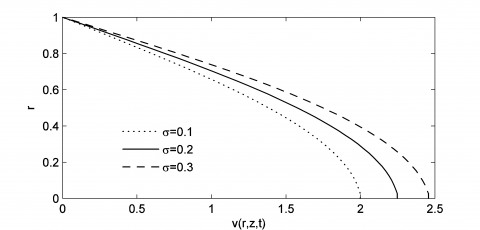(b)

Figure 5. The dimensionless axial velocity profile for different values of stenotic and aneurysm

Figure 6 provides the axial velocity pattern of the artery against different tapered angles of aneurysm and stenotic. Figure 6 pointed on this fact that the axial velocity inside the artery has an increasing pattern against the increasing angle. Also, the result of non-tapered artery is located between the convergent and divergent tapered arteries curves. These results agree closely with the observations of Lorenzini and Casalena  which they concluded that the blood flow velocity and recirculation are strongly affected by the stenotic slope. Figure 7 shows the effect of Prandtl number on the temperature distributions inside the artery. This figure shows that the heat transfer rate will decrease with increase in the Prandtl number. In other words, the rate of heat transferred from the artery to the blood decreases with increase in the Prandtl number.

## figure_6.pngFigure 6. The dimensionless axial velocity profile for different tapered angles

## figure_7.png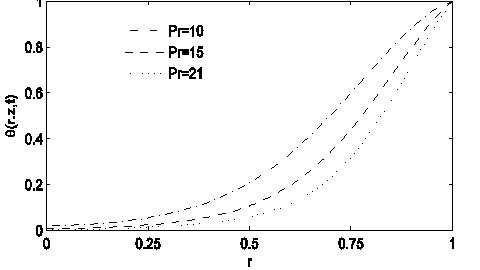Figure 7. The dimensionless temperature profile for different prandtl numbers

Figure 8 denotes the variation of Br against the dimensionless temperature profile. It can be seen that the temperature of crossing blood increases with increases in the Br value. Also, it can be seen that when Br increases the heat transfer decreases as the result of temperature increasing.

## figure_8.png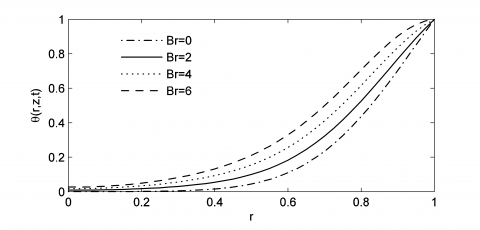Figure 8. The dimensionless temperature profile for different prandtl numbers

Figure 9 the effect of body acceleration (${{D}_{2}}$) on the non-Newtonian flow rate (blood) is described in Figure 9. Figure 9a declares that the blood flow is strongly up to the acceleration of the body. When ${{D}_{2}}$ is increased slowly, the maximum velocity develops sooner. Since the axial velocity of the blood flow and the volumetric rate are in a close relationship, the flow rate will increase with improvement in the body acceleration parameter. Figure 9b shows that the profiles of the flow rate which changes against the time. As a result, comparison between three different values of stenosis/aneurysms leads to this point; increase in the aneurysm value can result a significant enhance in the volumetric flow rate. (The graph shows the pulse behavior blood flow in different heartfelt periods).

## figure_9a.png(a)

## figure_9b.png(b)

Figure 9. The flow rate profile for different acceleration values

## figure_10a.png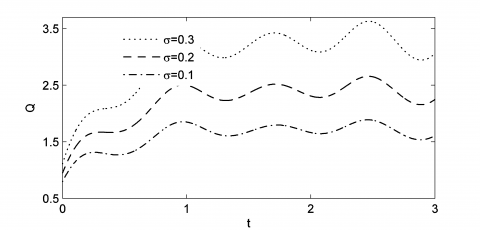(a)

## figure_10b.png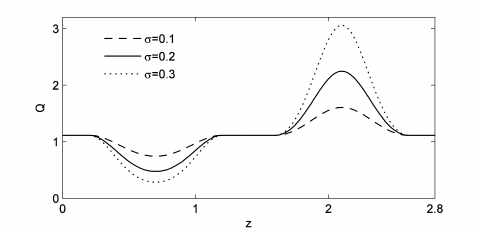(b)

Figure 10. The Flow rate profile for different values of aneurysm

Figure 10a shows the effect of body acceleration parameter on the blood flow inside the considered artery. As said before, the acceleration of body and the blood flow rate are directly related to each other, so, when the velocity increases, the flow rate increases too. Figure 10b describes the effect of aneurysms/ stenotic size on the blood flow against the position and place. When the aneurysms size increases the flow rate improves and consequently the blood velocity increases.

Figure 11 illustrates the effect of Weissenberg number (as a dimensionless number at different times) on dimensionless blood flow rate. In can be visible that the blood flow rate will reduce with the increasing Weissenberg number. As another result, the patterns or treatments of volumetric flow rates for the Weissenberg numbers are related to the geometry of the stenosis and aneurysms.

Figure 12 the effect of Reynolds number on the blood flow resistance is indicated in Figure 11. This figure illustrates that when the Reynolds number increases the resistance against the blood flow increases. In other words, the pressure drop enhances with the increasing velocity.

## figure_11.pngFigure 11. The flow rate profile for different Weissenberg number

## figure_12.pngFigure 12. The resistance to flow profile for different Reynolds number

Figure 13 tries to declare the impact of dimensionless Weissenberg number we at different times on dimensionless impedance. As shown in Figure 12, the enhancing Weissenberg number can create an important increase in impedance. Also, in can be understood that the volumetric flow rate plays an opposite role against the blood flow resistance.

Figure 14 indicates the flow resistance different time series for different Power law indexes. According to these results, increase in the Power law index leads to decrease in the blood flow resistance, or in other words, blood flow resistance decreases with the increasing n parameter.

## figure_13.png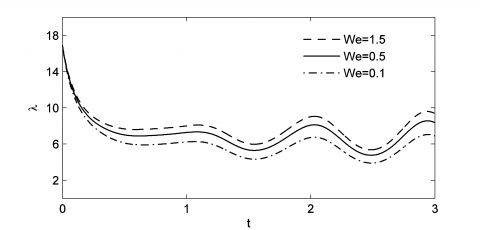Figure 13. The resistance impedance profile for different Weissenberg number

## figure_14.pngFigure 14. The resistance impedance profile for different power low index

Figure 15 the impact of Reynolds number on the wall shear stress is presented in Figure 15. This figure states that the wall shear stress can be increased when the related Reynolds number increases.

## figure_15.jpg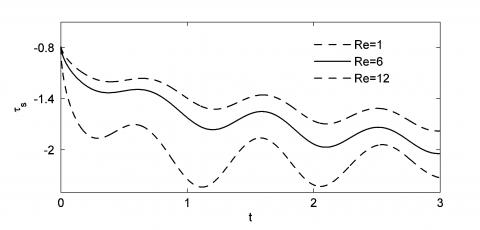Figure 15. The wall shear stress profile for different Reynolds number

## figure_16.jpgFigure 16. The wall shear stress profile for different values

Figure 16 based on the results of Figure 16 (at ‎$Z=2.1$), when the size of stenosis grows the wall shear stress increases. Furthermore, when the size of aneurysms increases the wall shear stress decreases.

Figure 17 points on the wall shear stress values for tapered and non-tapered arteries in geometry. As seen in this figure, the wall shear stress allays when the angle tapered increases. Also, it can be observed that the trend for non-tapered artery is located between the curves belong to the convergent and divergent arteries.

## figure_17.jpg.pngFigure 17. The wall shear stress profile for different tepered angles

## figure_18a.jpg(a)

## figure_18b.jpg(b)

## figure_18c.jpg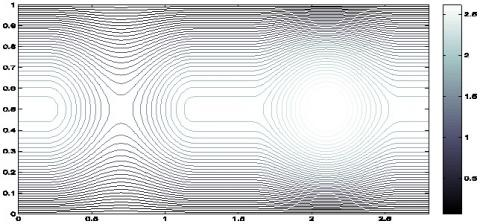(c)

## figure_18d.jpg(d)

## figure_18e.jpg(e)

## figure_18f.jpg(f)

Figure 18. Contour of velocity of blood flow

Figure 18 the patterns and behaviors of the blood flow for different values of‎ We, $\tau$, $\xi$, D2 and Re ‎are shown in Figure 18. Figure 18a shows a contour for some fixed parameters. By comparing plans a and b we found that when the Weissenberg number increases, the power of blood decreases. Besides, when the size of stenosis increases the blood flow velocity reduces and in the case of aneurysms, the velocity increases with increase in the height of aneurysms (comparing plans (a) and (c)). Furthermore, this comparison shows that in the non-tapered artery (left side) a small vortex is formed. In the systole phase the behavior is a little different from the diastolic phase. The effect of acceleration parameter on the blood flow is visible in the comparison view between plans (a) and (e). As said before, the flow velocity will increase with an improving acceleration. The effect of Reynolds number can be seen in plan (f). Also, when the Reynolds number is increased the flow rate on the arterial axis allays (the blood flow is reduced near the artery walls).

The two dimensional temperature distributions for some specific parameters are shown in Figure 19. The plan (a) has been compared to other plans in as a function of $\tau$, Br, We and sigma. As seen in comparison of plans (a) and (b), the temperature reduces when we have an increase the amount of stenosis and aneurysm. As another result, the increase in Brinkman and Weissenberg numbers leads to some positive and negative effects on the temperature values, reflectively (plans (a), (c) and (d)). The comparison between plans (a), (e) and (f) describes the effects of taper angle (divergent and convergent arteries) on the temperature profiles.

## figure_19a.jpg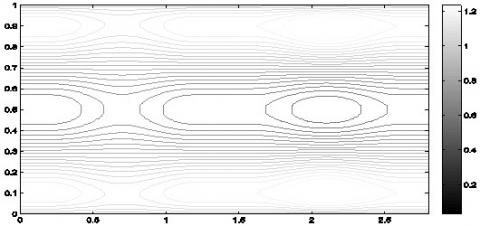(a)

## figure_19b.jpg(b)

## figure_19c.jpg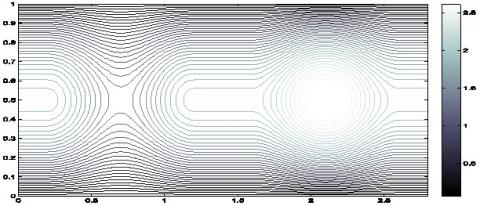(c)

## figure_19d.jpg(d)

## figure_19e.jpg(e)

## figure_19f.jpg(f)

Figure 19. The contour of temperature of blood flow

7. Conclusion

In this investigation the blood flow patters and the effects of heat transfer between the wall of arteries and the flow on the blood characteristics have been studied. The mentioned parameters on a cross section area of the arteries in the axial direction have been analyzed in different situations. The velocity and temperature distributions are investigated as two main affective parameters on the blood flow. The Blood flow is assumed to have some conditions near the real state such as pulsed, nonlinear, layered and unstable and flowing through an elastic wall. The comparison between the present simulation and previous works shows a good agreement which proves the accuracy of the results. We also provided some comparisons between our results and those of Lorenzini and Casalena  who analyzed the blood flow in a coronary artery, affected by different stenotic shapes, via a CFD code. The results prove that when the Prandtl and Brinkman numbers increase, the heat transfer between the blood and the artery walls and the temperature values on the profiles {improve}. By comparing the elastic and the rigid walls, it can be seen that the axial velocity of elastic wall is less than the axial velocity regarding the rigid wall. Furthermore, when the aneurysm size is increased the axial velocity and the flow rate will be increased.

Nomenclature
 Br Brinkman number $C_p$ Specific heat fp Pulse frequency kr Oscillation parameter L Finite length of the arterial segment $l_0$ Length of the stenosis n Power law index p pressure Pr Prandtl number Q Rate of flow R(z) Radius of the nonstenotic Re Reynolds number S Extra stress tensor t time T Temperature u Radial velocity $U_0$ Average velocity v Axial velocity We Weissenberg number Greek symbols △k Radial direction $\tau_s$ Wall shear sterss $\sigma$ Critical heigh µ Viscosity $\Lambda$ Resistive impedance $\varphi$ Phase angle Subscripts i j k
References

 Mortazavinia Z, Zare A, Mehdizadeh A. (2012). Effects of renal artery stenosis on realistic model of abdominal aorta and renal arteries incorporating fluid-structure interaction and pulsatile non-Newtonian blood flow. Applied Mathematics and Mechanics 33(2): 165-176. http://dx.doi.org/10.1007/s10483-012-1541-6

 Mandal PK. (2005). An unsteady analysis of non-Newtonian blood flow through tapered arteries with a stenosis. International Journal of Non-Linear Mechanics 40(1): 151-164. https://doi.org/10.1016/j.ijnonlinmec.2004.07.007

 Haghighi AR, Pralhad RN. (2009). Mathematical modelling of blood flows under the effects of body forces and magnetism on human body. International Journal of Biomedical Engineering and Technology 2(4): 295-302. http://dx.doi.org/10.1504/IJBET.2009.027794

 Mekheimer KS, El Kot MA. (2008). The micropolar fluid model for blood flow through a tapered artery with a stenosis. Acta Mechanica Sinica 24(6): 637-644. http://dx.doi.org/10.1007/s10409-008-0185-7

 Pontrelli G. (1998). Pulsatile blood flow in a pipe. Computers & Fluids 27(3): 367-380. https://doi.org/10.1016/S0045-7930(97)00041-8

 Lee JS, Fung YC. (1970). Flow in locally constricted tubes at low Reynolds numbers. Journal of Applied Mechanics 37(1): 9-16. https://doi.org/10.1115/1.3408496

 Azuma T, Fukushima T. (1976). Flow patterns in stenotic blood vessel models. Biorheology 13(6): 337-355. https://doi.org/10.3233/BIR-1976-13602

 Liu GT, Wang XJ, Ai BQ, Liu LG. (2004). Numerical study of pulsating flow through a tapered artery with stenosis. Chinese Journal of Physics 42(4): 401-409. https://doi.org/10.6122/CJP

  Ismail Z, Abdullah I, Mustapha N, Amin N. (2008). A power-law model of blood flow through a tapered overlapping stenosed artery. Applied Mathematics and Computation 195(2): 669-680.‏ https://doi.org/10.1016/j.amc.2007.05.014

 Akbar NS, Nadeem S. (2014). Carreau fluid model for blood flow through a tapered artery with a stenosis. Ain Shams Engineering Journal 5(4): 1307-1316.‏ https://doi.org/10.1016/j.asej.2014.05.010

 Zaman A, Ali N, Sajid M, Hayat T. (2015). Effects of unsteadiness and non-Newtonian rheology on blood flow through a tapered time-variant stenotic artery. AIP Advances 5(3): 037129.‏ https://doi.org/10.1063/1.4916043

 Rathod VP, Tanveer S, Rani IS, Rajput GG. (2006). Pulsatile flow of blood with periodic body acceleration and magnetic field through an exponentially diverging vessel. Ultra Sci. Phys. Sci. 18: 417-426.‏

 Burton RR, Leverett JS, Michaelson ED. (1974). Man at high sustained Gz acceleration: A review, Aerospace Medicine 45(10): 1115-1136.

 Chakravarty S, Sannigrahi AK. (1998). An analytical estimate of the flow-field in a porous stenotic artery subject to body acceleration. International Journal of Engineering Science 36(10): 1083-1102.‏ https://doi.org/10.1016/S0020-7225(98)00009-3

 Haghighi AR, Asl MS. (2015). Mathematical modeling of micropolar fluid flow through an overlapping arterial stenosis. International Journal of Biomathematics 8(04): 1550056.‏ https://doi.org/10.1142/s1793524515500564

 Zaman A, Ali N, Bég OA. (2016). Numerical simulation of unsteady micropolar hemodynamics in a tapered catheterized artery with a combination of stenosis and aneurysm. Medical & Biological Engineering & Computing 54(9): 1423-1436.‏ https://doi.org/10.1007/s11517-015-1415-3

 Shit GC, Majee S. (2015). Pulsatile flow of blood and heat transfer with variable viscosity under magnetic and vibration environment. Journal of Magnetism and Magnetic Materials 388: 106-115.‏ http://dx.doi.org/10.1016/j.jmmm.2015.04.026

 Eldabe NT, Moatimid GM, Hassan MA, Mostapha DR. (2016). Effects of partial slip on peristaltic flow of a Sisko fluid with mild stenosis through a porous medium. Appl. Math. Inf. Sci. 10(2): 673-687.‏ http://dx.doi.org/10.18576/amis/100227

  Zaman A, Ali N, Bég OA, Sajid M. (2016). Heat and mass transfer to blood flowing through a tapered overlapping stenosed artery. International Journal of Heat and Mass Transfer 95: 1084-1095.‏ https://doi.org/10.1016/j.ijheatmasstransfer.2015.12.073

 Mekheimer KS, Haroun MH, El Kot MA. (2012). Influence of heat and chemical reactions on blood flow through an anisotropically tapered elastic arteries with overlapping stenosis. Appl. Math 6(2): 281-292.‏

 Mekheimer KS, El Kot MA. (2012). Mathematical modelling of unsteady flow of a Sisko fluid through an anisotropically tapered elastic arteries with time-variant overlapping stenosis. Applied Mathematical Modelling 36(11): 5393-5407.‏ https://doi.org/10.1016/j.apm.2011.12.051

 Sankar DS, Lee U. (2011). Nonlinear mathematical analysis for blood flow in a constricted artery under periodic body acceleration. Communications in Nonlinear Science and Numerical Simulation 16(11): 4390-4402.‏ https://doi.org/10.1016/j.cnsns.2011.03.020

 Chakravarty S, Mandal PK. (2009). Effect of heat and mass transfer on non-Newtonian flow–Links to atherosclerosis. International Journal of Heat and Mass Transfer 52(25-26): 5719-5730.‏ https://doi.org/10.1016/j.ijheatmasstransfer.2009.04.040

 Victor SA, Shah VL. (1975). High transfer to blood flowing in a tube. Biorheology 12: 361. https://doi.org/10.3233/BIR-1975-12606

 Zaman A, Ali N, Bég OA. (2016). Numerical study of unsteady blood flow through a vessel using Sisko model. Engineering Science and Technology, an International Journal 19(1): 538-547.‏ https://doi.org/10.1016/j.jestch.2015.09.013

 Lorenzini G, Casalena E. (2008). CFD analysis of pulsatile blood flow in an atherosclerotic human artery with eccentric plaques. Journal of Biomechanics 41(9): 1862-1870. https://doi.org/10.1016/j.jbiomech.2008.04.009

 Haghighi AR, Kabdool AA, Asl MS. (2016). Numerical investigation of pulsatile blood flow in stenosed artery. International Journal of Applied and Computational Mathematics 2(4): 649-662.‏ http://dx.doi.org/10.1007/s40819-015-0084-0

 Haghighi AR, Asl MS, Kiyasatfar M. (2015). Mathematical modeling of unsteady blood flow through elastic tapered artery with overlapping stenosis. Journal of the Brazilian Society of Mechanical Sciences and Engineering 37(2): 571-578.‏ https://doi.org/10.1007/s40430-014-0206-3

 Haghighi AR, Aliashrafi N. (2018). Mathematical modeling of pulsatile blood flow and heat transfer under magnetic and vibrating environment. International Journal of Heat and Technology 36(3): 783-790. https://doi.org/10.18280/ijht.360302

 Mabood F, Ibrahim ShM, Lorenzini G, Lorenzin E. (2017). Radiation effects on Williamson nanofluid flow over a heated surface with magnetohydrodynamics. International Journal of Heat and Technology 35(1): 196-204. http://dx.doi.org/10.18280/ijht.350126

 Haghighi AR, Chalak SA. (2017). Mathematical modeling of blood flow through a stenosed artery under body acceleration. Journal of the Brazilian Society of Mechanical Sciences and Engineering 39(7): 2487-2494. http://dx.doi.org/10.1007/s40430-017-0716-x

 Mustapha N, Mandal PK, Johnston PR, Amin N. (2010). A numerical simulation of unsteady blood flow through multi-irregular arterial stenoses. Applied Mathematical Modelling 34(6): 1559-1573.‏ https://doi.org/10.1016/j.apm.2009.09.008

 Amsden AA, Harlow FH. (1970). The SMAC method: A numerical technique for calculating incompressible fluid flows. LA--4370. Los Alamos Scientific Laboratory Report LA-4370. United States.

 Markham G, Proctor MV. (1983). Modifications to the two-dimensional incompressible fluid flow code ZUNI to provide enhanced performance. C.E.G.B. Report TPRD/L/0063/M82. Leatherhead, England.